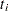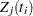Example 54.13 Time Dependent Cox Model

This example uses the same Myeloma data set as in Time Independent Cox Model, and illustrates the fitting of a time dependent Cox model. The following statements generate the data set once again:

data Myeloma;
input Time Vstatus LogBUN HGB Platelet Age LogWBC Frac
LogPBM Protein SCalc;
label Time='survival time'
datalines;
1.25  1  2.2175   9.4  1  67  3.6628  1  1.9542  12  10
1.25  1  1.9395  12.0  1  38  3.9868  1  1.9542  20  18
2.00  1  1.5185   9.8  1  81  3.8751  1  2.0000   2  15

... more lines ...

77.00  0  1.0792  14.0  1  60  3.6812  0  0.9542   0  12
;

To modelas a function of the survival time, you can relate timeto covariates by using this formula:For illustrational purposes, only three explanatory variables, LOGBUN, HBG, and PLATELET, are used in this example.

Sincedepends on, every term in the summation ofis a product of the current timeand all observations that are in the risk set. You can use the JOINTMODEL option, as in the last example, or you can modify the input data set such that every row contains not only the current observation but also all observations that are in the corresponding risk set. When you construct the log likelihood for each observation, you have all the relevant data at your disposal.

The following statements illustrate how you can create a new data set with different risk sets at different rows:

title 'Cox Model with Time Dependent Covariates';
proc sort data = Myeloma;
by descending time;
run;

data _null_;
set Myeloma nobs=_n;
call symputx('N', _n);
stop;
run;

ods select none;
proc freq data=myeloma;
tables time / out=freqs;
run;
ods select all;

proc sort data = freqs;
by descending time;
run;

data myelomaM;
set myeloma;
ind = _N_;
run;

data myelomaM;
merge myelomaM freqs(drop=percent); by descending time;
retain stop;
if first.time then do;
stop = _n_ + count - 1;
end;
run;

%macro array(list);
%global mcmcarray;
%let mcmcarray = ;
%do i = 1 %to 32000;
%let v = %scan(&list, &i, %str( ));
%if %nrbquote(&v) ne %then %do;
array _&v[&n];
%let mcmcarray = &mcmcarray array _&v[&n] _&v.1 - _&v.&n%str(;);
do i = 1 to stop;
set myelomaM(keep=&v) point=i;
_&v[i] = &v;
end;
%end;
%else %let i = 32001;
%end;
%mend;

data z;
set myelomaM;
%array(logbun hgb platelet);
drop vstatus logbun hgb platelet count stop;
run;

data myelomaM;
merge myelomaM z; by descending time;
run;

The data set MyelomaM contains 65 observations and 209 variables. For each observation, you see added variables stop, _logbun1 through _logbun65, _hgb1 through _hgb65, and _platelet1 through _platelet65. The variable stop indicates the number of observations that are in the risk set of the current observation. The rest are transposed values of model covariates of the entire data set. The data set contains a number of missing values. This is due to the fact that only the relevant observations are kept, such as _logbun1 to _logbunstop. The rest of the cells are filled in with missing values. For example, the first observation has a unique survival time of 92 and stop is 1, making it a risk set of itself. You see nonmissing values only in _logbun1, _hgb1, and _platelet1.

The following statements fit the Cox model by using PROC MCMC:

proc mcmc data=myelomaM outpost=outi nmc=50000 ntu=3000 seed=17
missing=ac;
ods select PostSummaries PostIntervals;
array beta;
&mcmcarray
parms (beta:) 0;
prior beta: ~ normal(0, prec=1e-6);

b = (beta1 + beta2 * time) * logbun +
(beta3 + beta4 * time) * hgb +
(beta5 + beta6 * time) * platelet;
S = 0;
do i = 1 to stop;
S = S + exp( (beta1 + beta2 * time) * _logbun[i] +
(beta3 + beta4 * time) * _hgb[i] +
(beta5 + beta6 * time) * _platelet[i]);
end;
loglike = vstatus * (b - log(S));

model general(loglike);
run;

Note that the option MISSING= is set to AC. This is due to missing cells in the input data set. You must use this option so that PROC MCMC retains observations that contain missing values.

The macro variable &mcmcarray is defined in the earlier part in this example. You can use a %put statement to print its value:

%put &mcmcarray;

This statement prints the following:

array _logbun _logbun1 - _logbun65; array _hgb _hgb1 - _hgb65; array
_platelet _platelet1 - _platelet65;

The macro uses the ARRAY statement to allocate three arrays, each of which links their corresponding data set variables. This makes it easier to reference these data set variables in the program. The PARMS statement puts all the parameters in the same block. The PRIOR statement gives them normal priors with large variance. The symbol b is the regression term, and S is cumulatively added from 1 to stop for each observation in the DO loop. The symbol loglike completes the construction of log likelihood for each observation and the MODEL statement completes the model specification.

Posterior summary and interval statistics are shown in Output 54.13.1.

Output 54.13.1 Summary Statistics on Cox Model with Time Dependent Explanatory Variables and Ties in the Survival Time, Using PROC MCMC
 Cox Model with Time Dependent Covariates

The MCMC Procedure

Posterior Summaries
Parameter N Mean Standard
Deviation
Percentiles
25% 50% 75%
beta1 50000 3.2397 0.8226 2.6835 3.2413 3.7830
beta2 50000 -0.1411 0.0471 -0.1722 -0.1406 -0.1092
beta3 50000 -0.0369 0.1017 -0.1064 -0.0373 0.0315
beta4 50000 -0.00409 0.00360 -0.00656 -0.00408 -0.00167
beta5 50000 0.3548 0.7359 -0.1634 0.3530 0.8445
beta6 50000 -0.0417 0.0359 -0.0661 -0.0423 -0.0181

Posterior Intervals
Parameter Alpha Equal-Tail Interval HPD Interval
beta1 0.050 1.6399 4.8667 1.6664 4.8752
beta2 0.050 -0.2351 -0.0509 -0.2294 -0.0458
beta3 0.050 -0.2337 0.1642 -0.2272 0.1685
beta4 0.050 -0.0111 0.00282 -0.0112 0.00264
beta5 0.050 -1.0317 1.8202 -1.0394 1.8100
beta6 0.050 -0.1107 0.0295 -0.1122 0.0269

You can also use the option JOINTMODEL to get the same inference and avoid transposing the data for every observation:

proc mcmc data=myelomaM outpost=outa nmc=50000 ntu=3000 seed=17 jointmodel;
ods select none;
array beta;      array timeA[&n];      array vstatusA[&n];
array logbunA[&n];  array hgbA[&n];       array plateletA[&n];
array stopA[&n];    array bZ[&n];         array S[&n];

begincnst;
timeA[ind]=time;          vstatusA[ind]=vstatus;
logbunA[ind]=logbun;      hgbA[ind]=hgb;
plateletA[ind]=platelet;  stopA[ind]=stop;
endcnst;

parms (beta:) 0;
prior beta: ~ normal(0, prec=1e-6);

jl = 0;
do i = 1 to &n;
v1 = beta1 + beta2 * timeA[i];
v2 = beta3 + beta4 * timeA[i];
v3 = beta5 + beta6 * timeA[i];
bZ[i] = v1 * logbunA[i] + v2 * hgbA[i] + v3 * plateletA[i];

/* sum over risk set without considering ties in time. */
S[i] = exp(bZ[i]);
if (i > 1) then do;
do j = 1 to (i-1);
b1 = v1 * logbunA[j] + v2 * hgbA[j] + v3 * plateletA[j];
S[i] = S[i] + exp(b1);
end;
end;
end;

/* make correction to the risk set due to ties in time. */
do i = 1 to &n;
if(stopA[i] > i) then do;
v1 = beta1 + beta2 * timeA[i];
v2 = beta3 + beta4 * timeA[i];
v3 = beta5 + beta6 * timeA[i];
do j = (i+1) to stopA[i];
b1 = v1 * logbunA[j] + v2 * hgbA[j] + v3 * plateletA[j];
S[i] = S[i] + exp(b1);
end;
end;
jl = jl + vstatusA[i] * (bZ[i] - log(S[i]));
end;
model general(jl);
run;

The multiple ARRAY statements allocate array symbols that are used to store the parameters (beta), the response (timeA), the covariates (vstatusA, logbunA, hgbA, plateletA, and stopA), and work space (bZ and S). The bZ and S arrays store the regression term and the risk set term for every observation. Programming statements in the BEGINCNST and ENDCNST statements input the response and covariates from the data set to the arrays.

Using the same technique shown in the example Time Independent Cox Model, the next DO loop calculates the regression term and corresponding S for every observation, pretending that there are no ties in time. This means that the risk set for observationinvolves only observationto. The correction terms are added to the corresponding S[i] in the second DO loop, conditional on whether the stop variable is greater than the observation count itself. The symbol jl cumulatively adds the log likelihood for the entire data set, and the MODEL statement specifies the joint log-likelihood function.

The following statements run PROC COMPARE and show that the output data set outa contains identical posterior samples as outi:

proc compare data=outi compare=outa;
ods select comparesummary;
var beta1-beta6;
run;

The results are not shown here.

The following statements use PROC PHREG to fit the same time dependent Cox model:

proc phreg data=Myeloma;
ods select PostSummaries PostIntervals;
model Time*VStatus(0)=LogBUN z2 hgb z3 platelet z4;
z2 = Time*logbun;
z3 = Time*hgb;
z4 = Time*platelet;
bayes seed=1 nmc=10000 outpost=phout;
run;

Coding is simpler than PROC MCMC. See Output 54.13.2 for posterior summary and interval statistics:

Output 54.13.2 Summary Statistics on Cox Model with Time Dependent Explanatory Variables and Ties in the Survival Time, Using PROC PHREG
 Cox Model with Time Dependent Covariates

The PHREG Procedure

Bayesian Analysis

Posterior Summaries
Parameter N Mean Standard
Deviation
Percentiles
25% 50% 75%
LogBUN 10000 3.2423 0.8311 2.6838 3.2445 3.7929
z2 10000 -0.1401 0.0482 -0.1723 -0.1391 -0.1069
HGB 10000 -0.0382 0.1009 -0.1067 -0.0385 0.0297
z3 10000 -0.00407 0.00363 -0.00652 -0.00404 -0.00162
Platelet 10000 0.3778 0.7524 -0.1500 0.3389 0.8701
z4 10000 -0.0419 0.0364 -0.0660 -0.0425 -0.0178

Posterior Intervals
Parameter Alpha Equal-Tail Interval HPD Interval
LogBUN 0.050 1.6059 4.8785 1.5925 4.8582
z2 0.050 -0.2361 -0.0494 -0.2354 -0.0492
HGB 0.050 -0.2343 0.1598 -0.2331 0.1603
z3 0.050 -0.0113 0.00297 -0.0109 0.00322
Platelet 0.050 -0.9966 1.9464 -1.1342 1.7968
z4 0.050 -0.1124 0.0296 -0.1142 0.0274